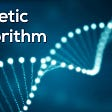# Core logic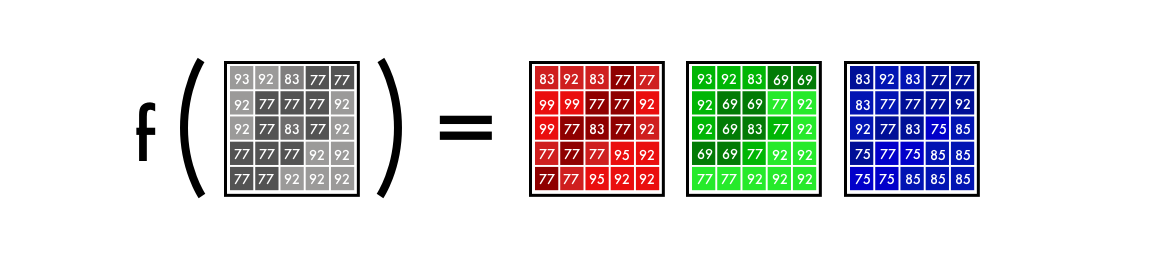f() is the neural network, [B&W] is our input, and [R],[G],[B] is our output.

# Alpha version

## Alpha version

`git clone https://github.com/emilwallner/Coloring-greyscale-images-in-Keras`
`cd Coloring-greyscale-images-in-Keras/floydhubfloyd init colornet`
`floyd init colornet`
`floyd run --data emilwallner/datasets/colornet/2:data --mode jupyter --tensorboard`
• We mounted a public dataset on FloydHub (which I’ve already uploaded) at the `data`directory with the below line:
`--dataemilwallner/datasets/colornet/2:data`
• We enabled Tensorboard with `--tensorboard`
• We ran the job in Jupyter Notebook mode with `--mode jupyter`
• If you have GPU credit, you can also add the GPU flag `--gpu`to your command. This will make it approximately 50x faster
`floydhub/Alpha version/working_floyd_pink_light_full.ipynb`
`model.fit(x=X, y=Y, batch_size=1, epochs=1)`
`# Get imagesimage = img_to_array(load_img('woman.png'))image = np.array(image, dtype=float)# Import map images into the lab colorspaceX = rgb2lab(1.0/255*image)[:,:,0]Y = rgb2lab(1.0/255*image)[:,:,1:]Y = Y / 128X = X.reshape(1, 400, 400, 1)Y = Y.reshape(1, 400, 400, 2)# Building the neural networkmodel = Sequential()model.add(InputLayer(input_shape=(None, None, 1)))model.add(Conv2D(8, (3, 3), activation='relu', padding='same', strides=2))model.add(Conv2D(8, (3, 3), activation='relu', padding='same'))model.add(Conv2D(16, (3, 3), activation='relu', padding='same'))model.add(Conv2D(16, (3, 3), activation='relu', padding='same', strides=2))model.add(Conv2D(32, (3, 3), activation='relu', padding='same'))model.add(Conv2D(32, (3, 3), activation='relu', padding='same', strides=2))model.add(UpSampling2D((2, 2)))model.add(Conv2D(32, (3, 3), activation='relu', padding='same'))model.add(UpSampling2D((2, 2)))model.add(Conv2D(16, (3, 3), activation='relu', padding='same'))model.add(UpSampling2D((2, 2)))model.add(Conv2D(2, (3, 3), activation='tanh', padding='same'))# Finish modelmodel.compile(optimizer='rmsprop',loss='mse')#Train the neural networkmodel.fit(x=X, y=Y, batch_size=1, epochs=3000)print(model.evaluate(X, Y, batch_size=1))# Output colorizationsoutput = model.predict(X)output = output * 128canvas = np.zeros((400, 400, 3))canvas[:,:,0] = X[:,:,0]canvas[:,:,1:] = outputimsave("img_result.png", lab2rgb(canvas))imsave("img_gray_scale.png", rgb2gray(lab2rgb(canvas)))`
`floyd run --data emilwallner/datasets/colornet/2:data --mode jupyter --tensorboard`

## Technical explanation

`X = rgb2lab(1.0/255*image)[:,:,0]Y = rgb2lab(1.0/255*image)[:,:,1:]`
`Y = Y / 128`
`output = model.predict(X)output = output * 128`
`canvas = np.zeros((400, 400, 3))canvas[:,:,0] = X[:,:,0]canvas[:,:,1:] = output`

## Takeaways from the Alpha version

• Reading research papers is challenging. Once I summarized the core characteristics of each paper, it became easier to skim papers. It also allowed me to put the details into a context.
• Starting simple is key. Most of the implementations I could find online were 2–10K lines long. That made it hard to get an overview of the core logic of the problem. Once I had a barebones version, it became easier to read both the code implementation, and also the research papers.
• Explore public projects. To get a rough idea for what to code, I skimmed 50–100 projects on colorization on Github.
• Things won’t always work as expected. In the beginning, it could only create red and yellow colors. At first, I had a Relu activation function for the final activation. Since it only maps numbers into positive digits, it could not create negative values, the blue and green spectrums. Adding a tanh activation function and mapping the Y values fixed this.
• Understanding > Speed. Many of the implementations I saw were fast but hard to work with. I chose to optimize for innovation speed instead of code speed.

# Beta version

## From feature extraction to color

`# Get imagesX = []for filename in os.listdir('../Train/'):    X.append(img_to_array(load_img('../Train/'+filename)))X = np.array(X, dtype=float)# Set up training and test datasplit = int(0.95*len(X))Xtrain = X[:split]Xtrain = 1.0/255*Xtrain#Design the neural networkmodel = Sequential()model.add(InputLayer(input_shape=(256, 256, 1)))model.add(Conv2D(64, (3, 3), activation='relu', padding='same'))model.add(Conv2D(64, (3, 3), activation='relu', padding='same', strides=2))model.add(Conv2D(128, (3, 3), activation='relu', padding='same'))model.add(Conv2D(128, (3, 3), activation='relu', padding='same', strides=2))model.add(Conv2D(256, (3, 3), activation='relu', padding='same'))model.add(Conv2D(256, (3, 3), activation='relu', padding='same', strides=2))model.add(Conv2D(512, (3, 3), activation='relu', padding='same'))model.add(Conv2D(256, (3, 3), activation='relu', padding='same'))model.add(Conv2D(128, (3, 3), activation='relu', padding='same'))model.add(UpSampling2D((2, 2)))model.add(Conv2D(64, (3, 3), activation='relu', padding='same'))model.add(UpSampling2D((2, 2)))model.add(Conv2D(32, (3, 3), activation='relu', padding='same'))model.add(Conv2D(2, (3, 3), activation='tanh', padding='same'))model.add(UpSampling2D((2, 2)))# Finish modelmodel.compile(optimizer='rmsprop', loss='mse')# Image transformerdatagen = ImageDataGenerator(        shear_range=0.2,        zoom_range=0.2,        rotation_range=20,        horizontal_flip=True)# Generate training databatch_size = 50def image_a_b_gen(batch_size):    for batch in datagen.flow(Xtrain, batch_size=batch_size):        lab_batch = rgb2lab(batch)        X_batch = lab_batch[:,:,:,0]        Y_batch = lab_batch[:,:,:,1:] / 128        yield (X_batch.reshape(X_batch.shape+(1,)), Y_batch)# Train modelTensorBoard(log_dir='/output')model.fit_generator(image_a_b_gen(batch_size), steps_per_epoch=10000, epochs=1)# Test imagesXtest = rgb2lab(1.0/255*X[split:])[:,:,:,0]Xtest = Xtest.reshape(Xtest.shape+(1,))Ytest = rgb2lab(1.0/255*X[split:])[:,:,:,1:]Ytest = Ytest / 128print model.evaluate(Xtest, Ytest, batch_size=batch_size)# Load black and white imagescolor_me = []for filename in os.listdir('../Test/'):        color_me.append(img_to_array(load_img('../Test/'+filename)))color_me = np.array(color_me, dtype=float)color_me = rgb2lab(1.0/255*color_me)[:,:,:,0]color_me = color_me.reshape(color_me.shape+(1,))# Test modeloutput = model.predict(color_me)output = output * 128# Output colorizationsfor i in range(len(output)):        cur = np.zeros((256, 256, 3))        cur[:,:,0] = color_me[i][:,:,0]        cur[:,:,1:] = output[i]        imsave("result/img_"+str(i)+".png", lab2rgb(cur))`
`floyd run --data emilwallner/datasets/colornet/2:data --mode jupyter --tensorboard`

## Technical explanation

`for filename in os.listdir('/Color_300/Train/'):    X.append(img_to_array(load_img('/Color_300/Test'+filename)))`
`datagen = ImageDataGenerator(        shear_range=0.2,        zoom_range=0.2,        rotation_range=20,        horizontal_flip=True)`
`batch_size = 50def image_a_b_gen(batch_size):    for batch in datagen.flow(Xtrain, batch_size=batch_size):        lab_batch = rgb2lab(batch)        X_batch = lab_batch[:,:,:,0]        Y_batch = lab_batch[:,:,:,1:] / 128        yield (X_batch.reshape(X_batch.shape+(1,)), Y_batch)`
`model.fit_generator(image_a_b_gen(batch_size), steps_per_epoch=1, epochs=1000)`

## Takeaways

• Run a lot of experiments in smaller batches before you make larger runs. Even after 20–30 experiments, I still found mistakes. Just because it’s running doesn’t mean it’s working. Bugs in a neural network are often more nuanced than traditional programming errors. One of the more bizarre ones was my Adam hiccup.
• A more diverse dataset makes the pictures brownish. If you have very similar images, you can get a decent result without needing a more complex architecture. The trade-off is the network becomes worse at generalizing.
• Shapes, shapes, and shapes. The size of each image has to be exact and remain proportional throughout the network. In the beginning, I used an image size of 300. Halving this three times gives sizes of 150, 75, and 35.5. The result is losing half a pixel! This led to many “hacks” until I realized it’s better to use a power of two: 2, 8, 16, 32, 64, 256 and so on.
• Creating datasets: a) Disable the .DS_Store file, it drove me crazy. b) Be creative. I ended up with a Chrome console script and an extension to download the files. c) Make a copy of the raw files you scrape and structure your cleaning scripts.

# Full-version

• Manually adding small dots of color in a picture to guide the neural network (link)
• Find a matching image and transfer the coloring (learn more here and here)
• Residual encoder and merging classification layers (link)
• Merging hypercolumns from a classifying network (more detail here and here)
• Merging the final classification between the encoder and decoder (details here and here)
`# Get imagesX = []for filename in os.listdir('/data/images/Train/'):    X.append(img_to_array(load_img('/data/images/Train/'+filename)))X = np.array(X, dtype=float)Xtrain = 1.0/255*X#Load weightsinception = InceptionResNetV2(weights=None, include_top=True)inception.load_weights('/data/inception_resnet_v2_weights_tf_dim_ordering_tf_kernels.h5')inception.graph = tf.get_default_graph()embed_input = Input(shape=(1000,))#Encoderencoder_input = Input(shape=(256, 256, 1,))encoder_output = Conv2D(64, (3,3), activation='relu', padding='same', strides=2)(encoder_input)encoder_output = Conv2D(128, (3,3), activation='relu', padding='same')(encoder_output)encoder_output = Conv2D(128, (3,3), activation='relu', padding='same', strides=2)(encoder_output)encoder_output = Conv2D(256, (3,3), activation='relu', padding='same')(encoder_output)encoder_output = Conv2D(256, (3,3), activation='relu', padding='same', strides=2)(encoder_output)encoder_output = Conv2D(512, (3,3), activation='relu', padding='same')(encoder_output)encoder_output = Conv2D(512, (3,3), activation='relu', padding='same')(encoder_output)encoder_output = Conv2D(256, (3,3), activation='relu', padding='same')(encoder_output)#Fusionfusion_output = RepeatVector(32 * 32)(embed_input) fusion_output = Reshape(([32, 32, 1000]))(fusion_output)fusion_output = concatenate([encoder_output, fusion_output], axis=3) fusion_output = Conv2D(256, (1, 1), activation='relu', padding='same')(fusion_output)#Decoderdecoder_output = Conv2D(128, (3,3), activation='relu', padding='same')(fusion_output)decoder_output = UpSampling2D((2, 2))(decoder_output)decoder_output = Conv2D(64, (3,3), activation='relu', padding='same')(decoder_output)decoder_output = UpSampling2D((2, 2))(decoder_output)decoder_output = Conv2D(32, (3,3), activation='relu', padding='same')(decoder_output)decoder_output = Conv2D(16, (3,3), activation='relu', padding='same')(decoder_output)decoder_output = Conv2D(2, (3, 3), activation='tanh', padding='same')(decoder_output)decoder_output = UpSampling2D((2, 2))(decoder_output)model = Model(inputs=[encoder_input, embed_input], outputs=decoder_output)#Create embeddingdef create_inception_embedding(grayscaled_rgb):    grayscaled_rgb_resized = []    for i in grayscaled_rgb:        i = resize(i, (299, 299, 3), mode='constant')        grayscaled_rgb_resized.append(i)    grayscaled_rgb_resized = np.array(grayscaled_rgb_resized)    grayscaled_rgb_resized = preprocess_input(grayscaled_rgb_resized)    with inception.graph.as_default():        embed = inception.predict(grayscaled_rgb_resized)    return embed# Image transformerdatagen = ImageDataGenerator(        shear_range=0.4,        zoom_range=0.4,        rotation_range=40,        horizontal_flip=True)#Generate training databatch_size = 20def image_a_b_gen(batch_size):    for batch in datagen.flow(Xtrain, batch_size=batch_size):        grayscaled_rgb = gray2rgb(rgb2gray(batch))        embed = create_inception_embedding(grayscaled_rgb)        lab_batch = rgb2lab(batch)        X_batch = lab_batch[:,:,:,0]        X_batch = X_batch.reshape(X_batch.shape+(1,))        Y_batch = lab_batch[:,:,:,1:] / 128        yield ([X_batch, create_inception_embedding(grayscaled_rgb)], Y_batch)#Train model      tensorboard = TensorBoard(log_dir="/output")model.compile(optimizer='adam', loss='mse')model.fit_generator(image_a_b_gen(batch_size), callbacks=[tensorboard], epochs=1000, steps_per_epoch=20)#Make a prediction on the unseen imagescolor_me = []for filename in os.listdir('../Test/'):    color_me.append(img_to_array(load_img('../Test/'+filename)))color_me = np.array(color_me, dtype=float)color_me = 1.0/255*color_mecolor_me = gray2rgb(rgb2gray(color_me))color_me_embed = create_inception_embedding(color_me)color_me = rgb2lab(color_me)[:,:,:,0]color_me = color_me.reshape(color_me.shape+(1,))# Test modeloutput = model.predict([color_me, color_me_embed])output = output * 128# Output colorizationsfor i in range(len(output)):    cur = np.zeros((256, 256, 3))    cur[:,:,0] = color_me[i][:,:,0]    cur[:,:,1:] = output[i]    imsave("result/img_"+str(i)+".png", lab2rgb(cur))`
`floyd run --data emilwallner/datasets/colornet/2:data --mode jupyter --tensorboard`

## Technical Explanation

`inception = InceptionResNetV2(weights=None, include_top=True)inception.load_weights('/data/inception_resnet_v2_weights_tf_dim_ordering_tf_kernels.h5')inception.graph = tf.get_default_graph()`
`grayscaled_rgb = gray2rgb(rgb2gray(batch))embed = create_inception_embedding(grayscaled_rgb)`
`def create_inception_embedding(grayscaled_rgb):    grayscaled_rgb_resized = []    for i in grayscaled_rgb:        i = resize(i, (299, 299, 3), mode='constant')        grayscaled_rgb_resized.append(i)    grayscaled_rgb_resized = np.array(grayscaled_rgb_resized)    grayscaled_rgb_resized = preprocess_input(grayscaled_rgb_resized)    with inception.graph.as_default():        embed = inception.predict(grayscaled_rgb_resized)    return embed`
`yield ([X_batch, create_inception_embedding(grayscaled_rgb)], Y_batch)`
`model = Model(inputs=[encoder_input, embed_input], outputs=decoder_output)`
`fusion_output = RepeatVector(32 * 32)(embed_input) fusion_output = Reshape(([32, 32, 1000]))(fusion_output)fusion_output = concatenate([fusion_output, encoder_output], axis=3) fusion_output = Conv2D(256, (1, 1), activation='relu')(fusion_output)`

## Takeaways

• The research terminology was daunting. I spent three days googling for ways to implement the “fusion model” in Keras. Because it sounded complex, I didn’t want to face the problem. Instead, I tricked myself into searching for short cuts.
• I asked questions online. I didn’t have a single comment in the Keras slack channel and Stack Overflow deleted my questions. But, by publicly breaking down the problem to make it simple to answer, it forced me to isolate the error, taking me closer to a solution.
• Email people. Although forums can be cold, people care if you connect with them directly. Discussing color spaces over Skype with a researcher is inspiring!
• After delaying on the fusion problem, I decided to build all the components before I stitched them together. Here are a few experiments I used to break down the fusion layer.
• Once I had something I thought would work, I was hesitant to run it. Although I knew the core logic was okay, I didn’t believe it would work. After a cup of lemon tea and a long walk — I ran it. It produced an error after the first line in my model. But after four days, several hundred bugs and several thousand Google searches, “Epoch 1/22” appeared under my model.

# Next steps

• Implement it with another pre-trained model
• Try a different dataset
• Increase the network’s accuracy by using more pictures
• Build an amplifier within the RGB color space. Create a similar model to the coloring network, that takes a saturated colored image as input and the correct colored image as output.
• Implement a weighted classification
• Apply it to video. Don’t worry too much about the colorization, but make the switch between images consistent. You could also do something similar for larger images, by tiling smaller ones.
• For the alpha version, simply replace the `woman.jpg`file with your file with the same name (image size 400x400 pixels).
• For the beta and the full version, add your images to the `Test`folder before you run the FloydHub command. You can also upload them directly in the Notebook to the Test folder while the notebook is running. Note that these images need to be exactly 256x256 pixels. Also, you can upload all test images in color because it will automatically convert them into B&W.

--

--

--

## More from Emil Wallner

Internet-educated, indie researcher, and in residency at Google Arts & Culture

Love podcasts or audiobooks? Learn on the go with our new app.

## 13 Best Cities On The Planet To Get Hired As A Data Scientist## How Big Data Solutions Shape The Travel Industry## [Part 1] Evaluating Offline Handwritten Text Recognition: Which Machine Learning Model is the…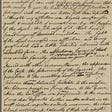## Working Towards Planetary Scale Location Insights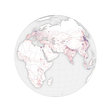## DataOps and the DataOps Manifesto## Vector tiles in QGIS 3.14## Emil Wallner

Internet-educated, indie researcher, and in residency at Google Arts & Culture

## Every Data Scientist Should Know This Design Principle## Ever Wondered How Your Hackathon Submission Is Reviewed? Learn It Here!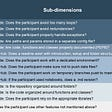## The Future of Data Visualization in Virtual Reality## A Simple way to optimise your program with Genetic Algorithm in Python: presenting the…